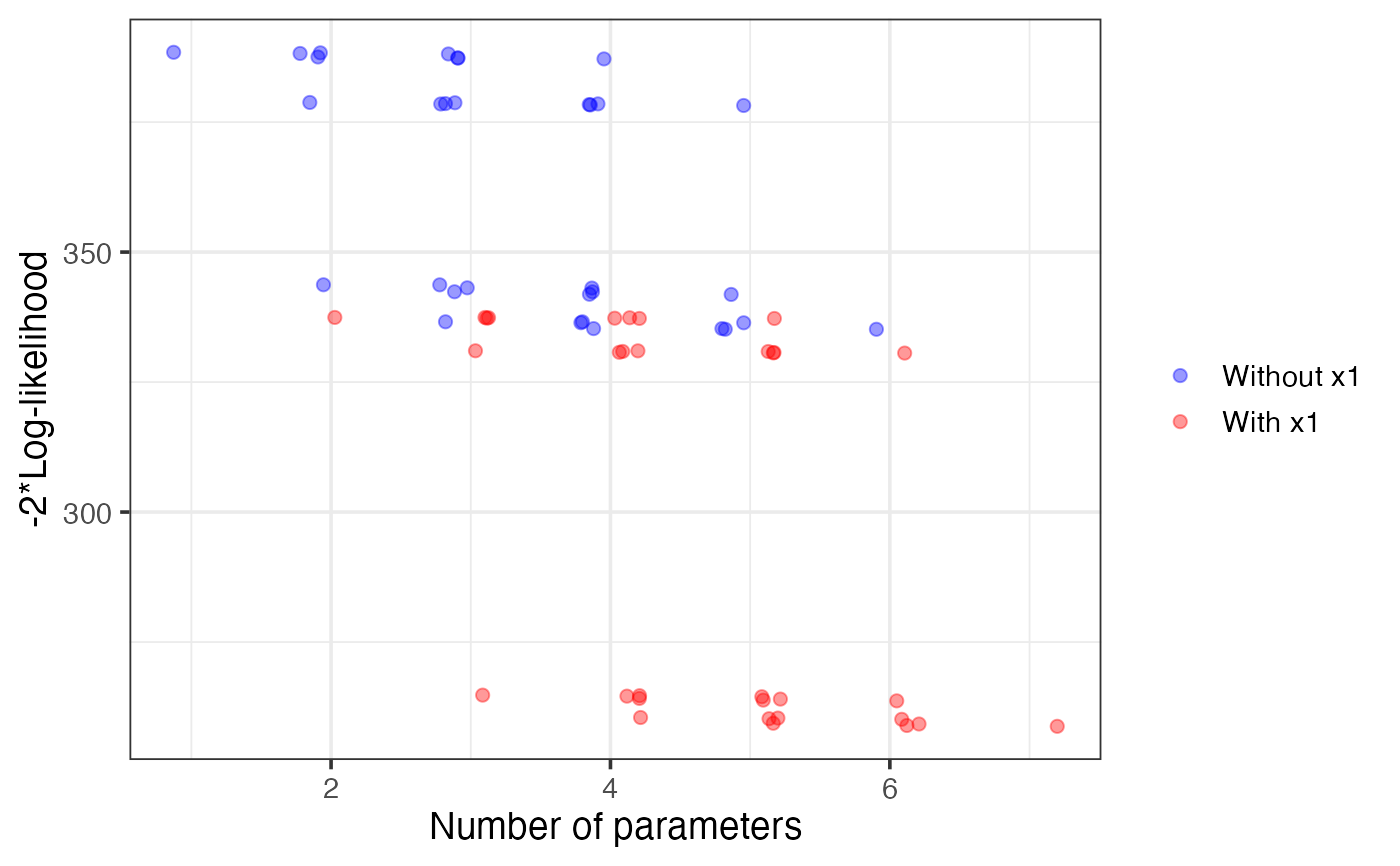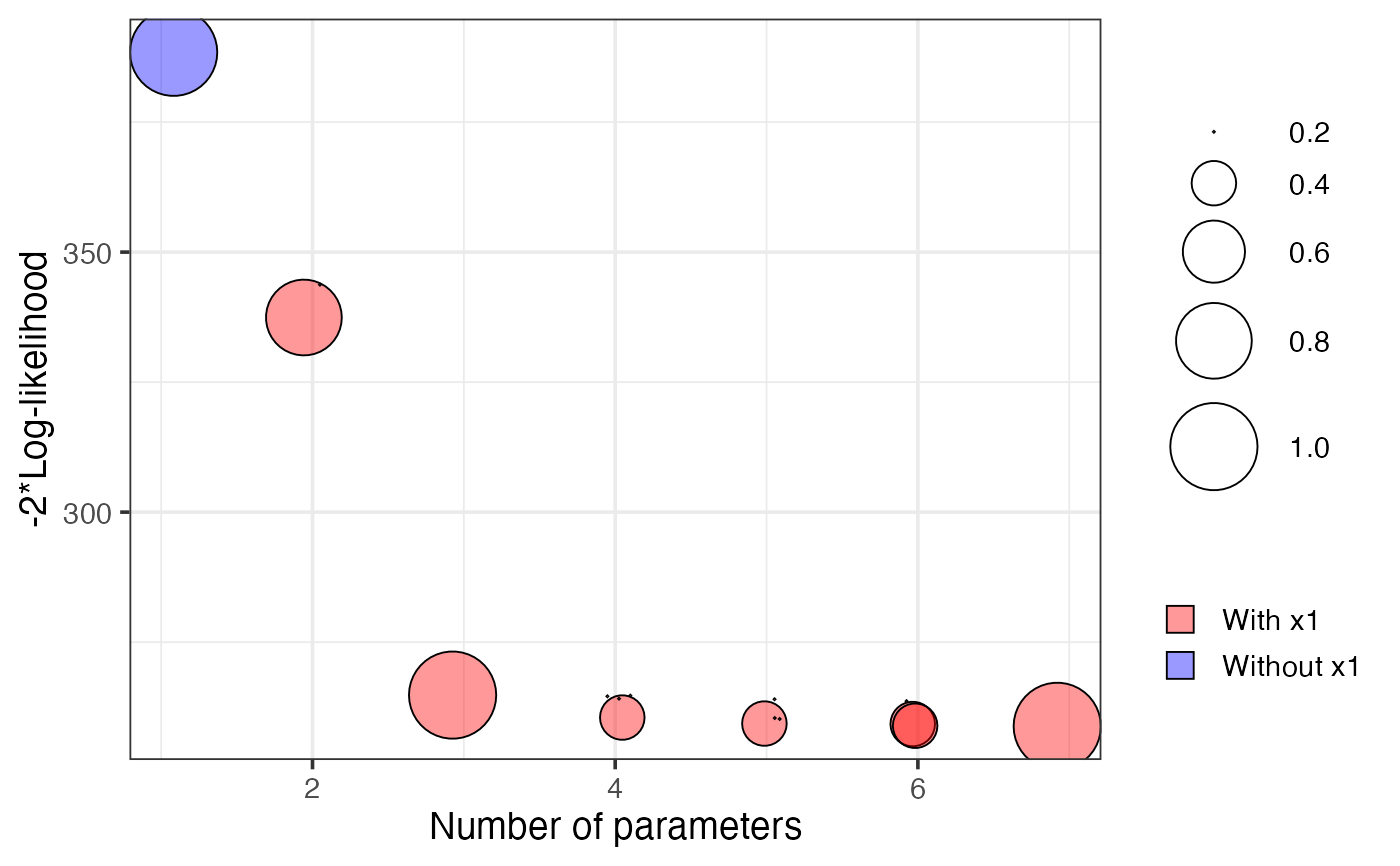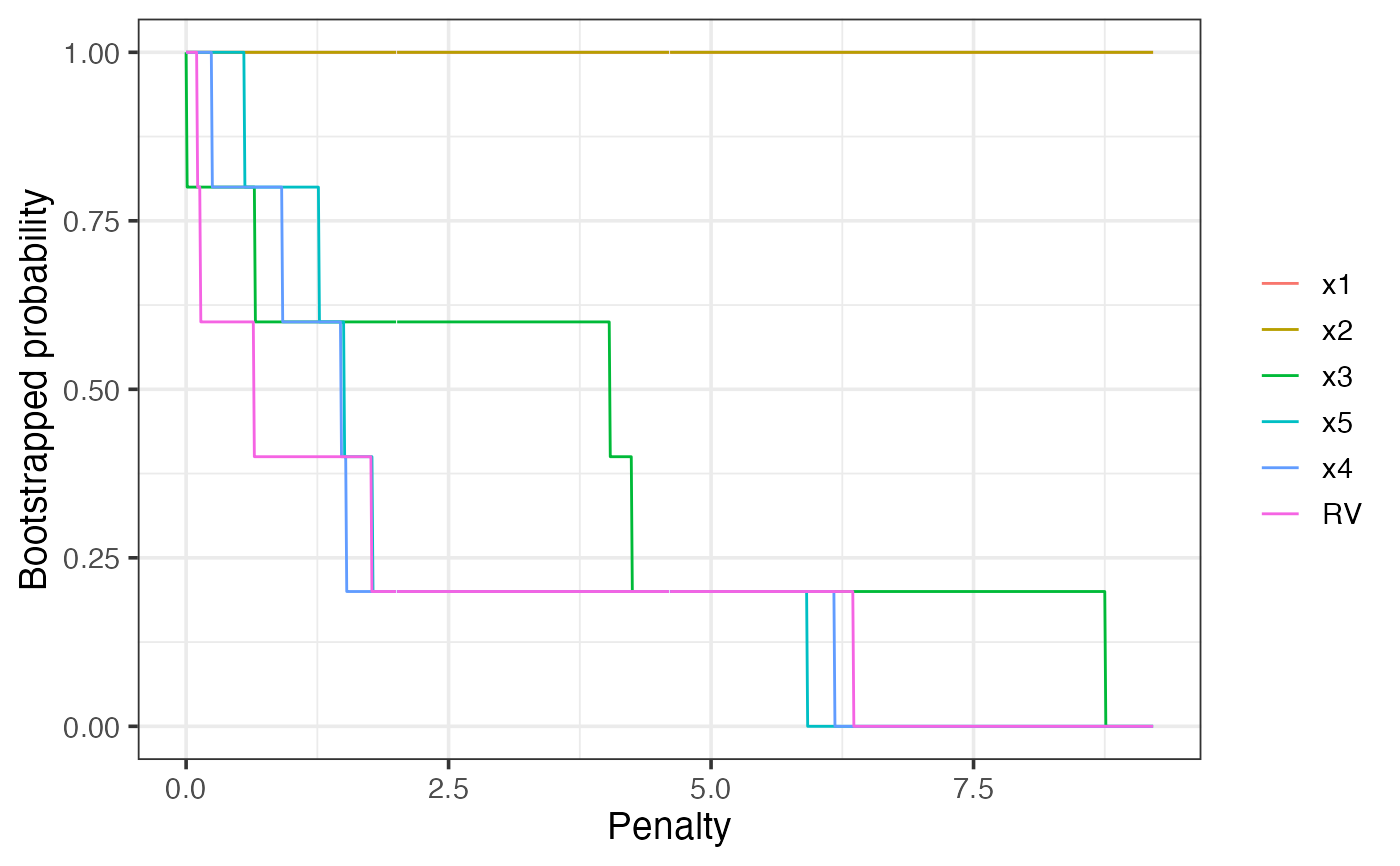A plot method to visualise the results of a vis object.

# S3 method for vis
plot(
x,
highlight,
interactive = FALSE,
classic = NULL,
tag = NULL,
shiny = FALSE,
nbest = "all",
which = c("vip", "lvk", "boot"),
width = 800,
height = 400,
fontSize = 12,
left = 50,
top = 30,
chartWidth = "60%",
chartHeight = "80%",
axisTitlesPosition = "out",
dataOpacity = 0.5,
options = NULL,
ylim,
legend.position = "right",
backgroundColor = "transparent",
text = FALSE,
min.prob = 0.4,
srt = 45,
max.circle = 15,
print.full.model = FALSE,
jitterk = 0.1,
seed = NULL,
...
)

## Arguments

x vis object, the result of vis the name of a variable that will be highlighted logical. If interactive=TRUE a googleVis plot is provided instead of the base graphics plot. Default is interactive=FALSE. logical. Depricated. If classic=TRUE a base graphics plot is provided instead of a googleVis plot. For now specifying classic will overwrite the default interactive behaviour, though this is likely to be removed in the future. Default NULL. Name tag of the objects to be extracted from a gvis (googleVis) object. The default tag for is NULL, which will result in R opening a browser window. Setting tag='chart' or setting options(gvis.plot.tag='chart') is useful when googleVis is used in scripts, like knitr or rmarkdown. Default FALSE. Set to TRUE when using in a shiny interface. maximum number of models at each model size that will be considered for the lvk plot. Can also take a value of "all" which displays all models (default). a vector specifying the plots to be output. Variable inclusion plots which="vip"; description loss against model size which="lvk"; bootstrapped description loss against model size which="boot". Width of the googleVis chart canvas area, in pixels. Default: 800. Height of the googleVis chart canvas area, in pixels. Default: 400. font size used in googleVis chart. Default: 12. space at left of chart (pixels?). Default: "50". space at top of chart (pixels?). Default: "30". googleVis chart area width. A simple number is a value in pixels; a string containing a number followed by % is a percentage. Default: "60%" googleVis chart area height. A simple number is a value in pixels; a string containing a number followed by % is a percentage. Default: "80%" Where to place the googleVis axis titles, compared to the chart area. Supported values: "in" - Draw the axis titles inside the the chart area. "out" - Draw the axis titles outside the chart area. "none" - Omit the axis titles. The transparency of googleVis data points, with 1.0 being completely opaque and 0.0 fully transparent. a list to be passed to the googleVis function giving complete control over the output. Specifying a value for options overwrites all other plotting variables. the y limits of the lvk and boot plots. the postion of the legend for classic plots. Default legend.position="right" alternatives include legend.position="top" and legend.position="bottom" The background colour for the main area of the chart. A simple HTML color string, for example: 'red' or '#00cc00'. Default: 'null' (there is an issue with GoogleCharts when setting 'transparent' related to the zoom window sticking - once that's sorted out, the default will change back to 'transparent') logical, whether or not to add text labels to classic boot plot. Default = FALSE. when text=TRUE, a lower bound on the probability of selection before a text label is shown. when text=TRUE, the angle of rotation for the text labels. Default = 45. determines the maximum circle size. Default = 15. logical, when text=TRUE this determines if the full model gets a label or not. Default=FALSE. amount of jittering of the model size in the lvk and boot plots. Default = 0.1. random seed for reproducible results further arguments (currently unused)

## Details

Specifying which = "lvk" generates a scatter plot where the points correspond to description loss is plot against model size for each model considered. The highlight argument is used to differentiate models that contain a particular variable from those that do not.

Specifying which = "boot" generates a scatter plot where each circle represents a model with a non-zero bootstrap probability, that is, each model that was selected as the best model of a particular dimension in at least one bootstrap replication. The area of each circle is proportional to the corresponding model's bootstrapped selection probability.

Mueller, S. and Welsh, A. H. (2010), On model selection curves. International Statistical Review, 78:240-256. doi: 10.1111/j.1751-5823.2010.00108.x

Murray, K., Heritier, S. and Mueller, S. (2013), Graphical tools for model selection in generalized linear models. Statistics in Medicine, 32:4438-4451. doi: 10.1002/sim.5855

Tarr G, Mueller S and Welsh AH (2018). mplot: An R Package for Graphical Model Stability and Variable Selection Procedures. Journal of Statistical Software, 83(9), pp. 1-28. doi: 10.18637/jss.v083.i09

vis

## Examples

n = 100
set.seed(11)
e = rnorm(n)
x1 = rnorm(n)
x2 = rnorm(n)
x3 = x1^2
x4 = x2^2
x5 = x1*x2
y = 1 + x1 + x2 + e
dat = data.frame(y,x1,x2,x3,x4,x5)
lm1 = lm(y~.,data=dat)
# \dontshow{
v1 = vis(lm1, B = 5, cores = 1, seed = 1)
plot(v1, highlight = "x1", which = "lvk")plot(v1, which = "boot")plot(v1, which = "vip")# }
if (FALSE) {
v1 = vis(lm1, seed = 1)
plot(v1, highlight = "x1", which = "lvk")
plot(v1, which = "boot")
plot(v1, which = "vip")
}